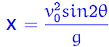# Projectile Motion: Pitcher throwing a ball to a catcher

## Homework Statement

determine the acute angle (in radians) of appropriate elevation in the throwing of a ball, if the initial velocity is 20 m / s, g=9.81 m/s^2 and the distance in x is 40m. The ball leaves the hand of the pitcher with an elevation equal to 1.8m and the catcher receives it at 1m.

## Homework Equations3. The Attempt at a Solution [/B]
I tried using the equation above, but then why gives me the values (Yo = 1.8m and Y=1m)?

#### Attachments

Last edited by a moderator:

## Answers and Replies

berkeman
Mentor
Welcome to the PF.In that equation, what is Vo? Is it total in the x direction, in the y direction, etc.? Can you show us your work using that equation?

Plus, it's best to get used to using all of the kinematic equations in solving problems like this, instead of trying to find one that seems to match the problem exactly. In this problem, for example, the takeoff and landing y distances are different, right?

Can you list more kinematic equations that apply to this problem, and try working the problem with those?

haruspex
Science Advisor
Homework Helper
Gold Member
2020 Award
I tried using the equation above
That equation is only for the case where launch and land are at the same height. It does not apply here.

•berkeman